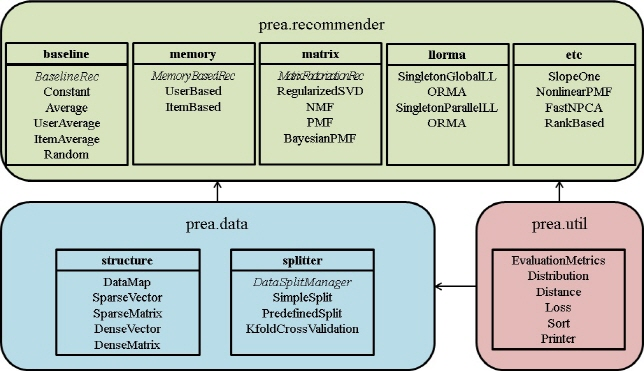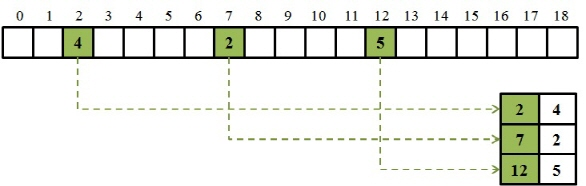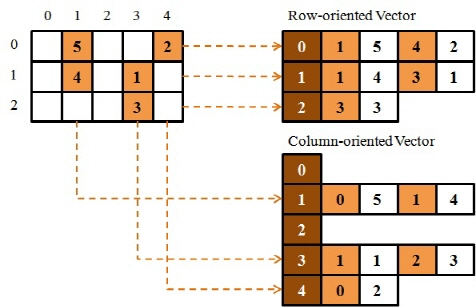﻿ PREA (Personalized Recommendation Algorithms Toolkit) :: Documentation
Last updated 2014-06-05

Documentation

# Class Structure Overview #The toolkit is composed of three big groups of packages. The first category (top in the diagram) contains recommendation algorithms, including constant baselines, memory-based methods, low-rank matrix factorization methods, and other state-of-the-art algorithms. Second one regards dataset management. It contains data structures, implementing both sparse and dense vectors and matrices, and data splitter supporting train/test split and crossvalidation. Last group implements useful utilities including evaluation metrics, statistical distributions, and other utility functions used in the toolkit.

# Data File (ARFF) #

PREA gets a sparse ARFF (Attribute-Relation File Format) file as an input. This file format is used in Weka, a widely-used data mining and machine learning toolkit.
The format starts with a declare of relation, with a statement of:
@RELATION [relation name]

Then, it consists of two sections: attribute definition and data. Attribute definition section is a set of following statement:
@ATTRIBUTE [attribute name] [attribute type]

Attribute type should be one of the following: NUMERIC, STRING, DATE, or a group of possible values with {}. For example,
@ATTRIBUTE score NUMERIC
@ATTRIBUTE name STRING
@ATTRIBUTE option {rock, scissor, paper}

After defining all the attributes used in the dataset, the data section starts with a statement:
@DATA

The data section can be one of the two possible formats: normal (dense) type and sparse type. The dense type is based on a comma-separated description of each dimension. This dense representation is, however, not supported in this toolkit, as most collaborative filtering dataset are extremely large and sparse. Instead, we use sparse representation of data section, like the following:
{1 X, 3 Y, 4 "class A"}
{2 W, 4 "class B"}

The example above means that the first row has three existing (non-zero in case of numeric type) values: "X" at index 1, "Y" at index 3, and "Class A" at index 4. In the second row, in a similar way, "W" is stored at index 2 and "class B" at index 4. In this way, if most of slots are missing or zeros, we can save significant amount of space by this sparse representation.

An original, full description about ARFF data format can be found here.

# Train/Test set Split #

Basically, inference is predicting about the current or future from the past. Thus, only past information are given to us for any kind of inference. When we are going to simulate this situation for evaluating recommender systems, we should take into account this effect. That is, it would be more accurate to real situation if we can restrict using rating information from the later points.

Gunawardana and Shani  introduced four strategies for splitting training and test set.
• For every available rating, try to predict it without using other ratings after the target rating is made. For instance, suppose the target rating is made at July 1, 2009. Then, we use a CF algorithm only with ratings after that date. This method is the most accurate, but it is too time-consuming as we need to check the date every time.
• Instead, we can just set a threshold date, saying July 1, 2009. Ratings after that threshold date are hidden for any prediction. In this way, we can save lots of time compared to the first option, losing some accuracy in the simulation.
• Unlike the above two methods, which use the exact date for threshold, the third one hides some portion of recent ratings from each user. Suppose we set the threshold as 20%. Then, the most recent 20% of ratings are separately hidden from each user. The threshold date may be different user by user.
• Lastly, we can ignore the timeline at all. Instead, randomly select some portion of ratings regardless of date, user, or item. This method is simplest and fastest to implement, but it may lose accuracy of simulating real situation.
We implemented train and test set split with the last option. Although it seems to lose accuracy in the respect of timeline simulation, we can reasonably assume that individual preference is not changing so fast in many applications. In this case, this method can be applied without much distortion. Also, this method is the only choice when the dataset does not provide date information for each rating. For general use of this toolkit, we decided to split the set without using date information. This is also adventageous when it comes to performance.
##### Split options in Prea
We provide three train/test set split methods listed below. Detailed format for selecting one of them can be found in Tutorial section.
• Simple split: This approach splits the whole dataset into simply two sets, train set and test set. Users can specify the proportion of test part as an agrument.
• Predefined split: If a predefined split is available, we can use the exactly same split again. This can be used for the purpose of verifying correctness of a newly implemented algorithm.
• K-fold Crossvalidation: The whole dataset is divided into K same-sized folds, and run expeirments K times with each Kth fold as test data and rest as train data. Prea shows evaluation result for each stage separately.
##### How to use predefined split
To ease repeated experiment, we provide a way to use predefined data split file. With this option, algorithm designers or testers may evaluate their algorithms by using exactly same dataset. To do this, the split file should be prepared properly first, as described below. You can use this file by giving an option "-s pred [file name]" as explained in Tutorial section. There are two ways of building this file, manually as well as automatically.
• Manual split: The format of split file is simple. For each line, each test point is stored. The format is "[User ID] [Item ID]", and those two IDs are separated by tab. Here is an example:
1 452
1 583
3 283
7 17
...
This manual split may be used if you want to use exactly same data split used in other toolkit or implementation.
• Automatic split: We provide automatic splitter file in prea.main package. By directly running Splitter.java, you can split the given dataset with designated train/test ratio. Here is the format as well as an example:
java Splitter [Input file name] [Test ratio] [(optional) -u] [(optional) -i]
java Splitter movielens_1M 0.2 -u
The options -u and -i additionally print user and item similarity files, respectively.

# Vector/Matrix Implementation #

PREA is implemented both with sparse and dense representation of vectors and matrices. Sparse ones are mainly used to represent dataset, while dense ones are for heavy matrix operations.
##### Sparse Vector/Matrix
Basically, rating matrix is generally extremely sparse, since most users do not provide their own ratings after purchase. On the other hand, very small number of users are passionate in giving feedback. For this reason, we have lots of users and items in the rating matrix, but only small pairs of (user, item) have actual rating value. Thus, we use sparse version of vector and matrix for representing these rating data in the toolkit.

Sparse vector/matrix is made with DataMap class, which is based on HashMap data structure provided by Java. For all pairs of with existing values, they are stored in a hash table in DataMap class. The following figure shows an example of sparse vector, with data in index 2, 7, and 12. As indicated in the figure, only three data points are stored in the hash table below in form. In this example, the sparse vector structure contains <2, 4>, <7, 2>, and <12, 5>.SparseMatrix is an array of SparseVector. We have both row-oriented and column-oriented vector, for faster access of rows and columns. Thus, when a new value is inserted or an existing value is deleted, both arrays should be maintained with the change. Note that when we edit a whole row or column, you should not make new row or column and replace the existing row or column with it. If you replace only row, for example, the column-oriented vector still has the old values, breaking consistency. We certainly do not provide methods for replacing a row or a column in this reason. This design is useful as we need to access each row for user-oriented task, as well as each column for item-oriented task. Also, this design simplifies transpose of a matrix, just by interchanging rows and columns each other. The following figure shows an example of sparse matrix.##### Dense Vector/Matrix
Dense vector or matrix is not used for representing the whole (or part of) raw dataset, as it is waste of memory space. However, some intermediate data can be dense even though the original dataset is extremely sparse. For instance, a user profile in a low-rank matrix (number of users by number of features) is dense. Representing this kind of intermediate matrix with sparse version can be inefficient, as sparse implementation requires at most twice of memory space since they use hash table. On the other hand, dense representation based on an array is efficient in memory usage as well as performing matrix operations. Thus, it may be a good idea to use dense representation for densely distributed vectors or matrices, to be used heavily with complex matrix operations such as inverse or Cholesky decomposition. However, converting from sparse one to dense one, or vice versa, is also time-consuming. In other words, we always have a trade-off between saving time with a proper representation for some task and lost time for conversion. A designer and programmer of new algorithm should take this trade-off into account for their implementation.

We used Universal Java Matrix Package (UJMP) for implementing dense vector/matrix. Provided operations with them are almost the same with those with sparse ones. For detailed description of provided methods, please refer to the API page.

# Test Interface #

For easier comparison of each CF algorithm, we provide a unified way of running each algorithm. A general order of running each class of algorithm is as follows. Each step provides an example with matrix factorization case.
• Declare an instance of class, to which the target algorithm belongs.
MatrixFactorization mf = new MatrixFactorization(rateMatrix, testMatrix, userCount, itemCount, maxValue, minValue, features, learningRate, regularizer, momentum, maxIter);
• Build a model with the given data and method code.
mf.buildModel(method);
• Predict ratings and compare them with ones in test set.
EvaluationMetrics MFresult = mf.evaluate(method);
• Print the result with various evaluation criteria.
System.out.println("All\t" + MFresult.getMAE() + "\t" + MFresult.getRMSE() + "\t" + MFresult.getHalflifeScore() + "\t" + (end-start));
Other algorithms also follow the same step, although some parameters may vary. One thing to note is that constant model and memory-based CF algorithms do not have the second step, building a model, as they are basically lazy methods, leaving prediction step by the moment of getting queries. In those models, we skip the second step, and do the prediction during evaluation step.

We can measure elapsed time for each step, with the following code:
long start = System.currentTimeMillis();
...
long end = System.currentTimeMillis();
This measures time taken between the two statements, in milliseconds. By calculating "end - start", we can get the elapsed time between them. It would be useful to measure both learning time (step 2) and evaluation time (step 3), separately.

# Common Functions #

We briefly describe common methods which can be applied anywhere inside the toolkit. For detailed description, please refer to the API page.
##### Statistical Distributions (Distribution.java)
Methods in this class implement random sampling from some statistical distributions, with given parameters.
• public static double normalRandom(double mean, double std)
Randomly sample 1 point from Normal Distribution with the given mean and standard deviation.
• public static double[] normalDistribution(double mean, double std, int count)
Randomly sample several points from Normal Distribution with the given mean and standard deviation.
• public static double gammaRandom(double alpha, double scale)
Randomly sample 1 point from Gamma Distribution with the given parameters.
• public static double[] gammaDistribution(double alpha, double scale, int count)
Randomly sample several points from Gamma Distribution with the given parameters.
• public static SparseMatrix wishartRandom(SparseMatrix scale, double df)
Randomly sample a matrix from Wishart Distribution with the given parameters.

##### Sorting (Sort.java)
Sort class includes several sorting methods which can be useful for implementing CF algorithms. Currently, we implemented a method for finding k-largest elements from an array and sorting them. For using a function in this class, you do not need to make an instance of the class, but just use each method by CFUtils.[function name].
• public static void kLargest(double[] array1, int[] array2, int first, int last, int k)
Find k largest elements from array1, and return them in sorted order. When their original index is given with array2, it will be also rearranged.
• public static void quickSort(int[] array, int first, int last, boolean increasingOrder)
Sort the given array. By setting increasingOrder differently, you can sort in either order.
• public static void quickSort(double[] array1, int[] array2, int first, int last, boolean increasingOrder)
Sort array1, and rearrange the original index in array2 as well.

##### Distance Measures (Distance.java)
This class helps to calculate several different measures of distancce between two vectors. Most of them are used in evaluation metrics class as well.
• public static double distanceNDCG(int[] uItemID, double[] relevance, int[] vItemID, double[] userScore)
Calculate NDCG score for a ranked list given the scores and relevance of items in the list.
• public static double distanceKendall(int[] uItemID, double[] uScore, int[] vItemID, double[] vScore, int n)
Calculate Kendall's Tau distance for two rankings.
• public static double distanceSpearman(int[] uItemID, double[] uScore, int[] vItemID, double[] vScore, int n)
Calculate Spearman distance for two rankings.
• public static void computeAverageRank(double[] score, double[] prb)
Calculate the average rank of each score with/without ties prb = (lowrank + (tie - 1) / 2) / (k + 1).

##### Printing functions (Printer.java)
We provide several printing functions for complex objects in a human-readable format. Currently, it includes printing an array, a matrix. Also, it supports printing in time format.
• public static void printArray(int[] A)
Print the array in tab-delimited format. We also have a version for double type.
• public static void printArray(double[][] A)
Print the matrix in tab-delimited format. We also have a version for 3D matrix.
• public static String printTime(long msType)
Print time stamp in long format into "DD days, HH:MM:SS.mmm" format.

# How to implement your own recommendation system #

We provide a skeleton code for potential authors of their own recommendation algorithm. In the package prea.recommender, you can find a class named "CustomRecommender". Following the instructions in that file leads you to build your own algorithm easily. You need to implement the follwing functions:
##### Constructors
• public CustomRecommender(int uc, int ic, double max, double min)
The default consturctor has 4 arguments, including user count, item count, max value, and min value. You may add your custom member variables to the class, then please make sure that they are correctly initialized in consturctors.
##### Model Builder
• public void buildModel(SparseMatrix rm)
Using the training data in "rm", you are supposed to write codes to learn your model here. If your method is memory-based one, you may leave the model as rateMatrix itself, simply by "rateMatrix = rm;". If your method is model-based algorithm, you may not need a reference to rateMatrix. (In this case, you may remove the variable "rateMatrix", just as matrix-factorization-based methods do in this toolkit.) Note that in any case train data in "rateMatrix" are read-only. You should not alter any value in it to guarantee proper operation henceforth.
##### Evaluation
• public EvaluationMetrics evaluate(SparseMatrix testMatrix)
To test your algorithm in the same manner with other algorithms in Prea, you need to implement this method according to the format. The method is designed to predict unseen ratings in testMatrix with your algorithm, and store your prediction in "predicted" matrix. What you need to is editing the part "double estimate = 0.0;" to put your prediction on item i by user u, instead of 0.0. From your model (model-based) or with your estimation method (memory-based) from rating matrix, If the estimation is not simple, you may make private methods to help the decision. Obviously again, you should not alter/add/remove any value in testMatrix during the evaluation process.

##### Unit Test
We provide a unit test module to help you verifying whether your implementation is correct. In the main method, you can make an instance of unit test module with your recommender by
CustomRecommender myRecommender = new CustomRecommender(userCount, itemCount, maxValue, minValue);
UnitTest u = new UnitTest(myRecommender, rateMatrix, testMatrix);

After you make the instance, simply call "check" method by
u.check();

The unit test module may print some warnings or errors based on verification result. If you get some errors, they should be fixed since they imply your implementation is illegal or incorrect. If you get some warnings, you may concern them and we recommend to investigate your code. If the unit test module does not find any problem, it will say so. We recommend to rerun with various parameters since some problems may occur occasionally.